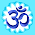# BHARDWAJ CLASSES

## Wave Optics Questions with Answers

### Wave Optics – Numerical Problems with Answers

1.    In Young’s double slit experiment using monochromatic light of wavelength λ, the intensity at a point on the screen where path difference is λ is k units. What is the intensity of light at a point where path difference is λ/3 ?
2.    In Young’s double slit experiment, angular width of a fringe is found to be 0.2° on a screen placed 1 m away. The wavelength of light used is 600 nm. What will be the angular width of the fringe, if the entire experimental apparatus is immersed in water ? Take refractive index of water = 4/3
3.    A Parallel beam of light of wavelength 500 nm falls on a narrow slit and the resulting diffraction pattern is observed on a screen 1 m away. It is observed that the first minima is at a distance of 2.5 mm from the centre of the screen, Find the width of slit.
4.    The distance between two slits in young interference experiment is 0.03 cm.  The 4th bright fringe is obtained at a distance of 1 cm from Central fringe on a screen placed at a distance of 1.5 m from slits. Calculate the wavelength of light used.
5.    A beam of light consisting of two wavelengths 800 nm and 600 nm is used to obtain the interference fringes in a Young's double slit experiment on a screen placed 1.4 m away, if the two slits are separated by 0.28 mm, Calculate the least distance from the central bright maximum where the bright fringes of two wavelengths coincide.
6.    Assume that the light of wavelength 6000  Å is coming from a star. Find the limit of resolution of a telescope whose objective has a diameter of 250 cm.
7.    Two slits are made 1 mm apart and the screen is placed 1 m away. What should be the width of each slit to obtain 10 maximum of the double slit pattern within the central maximum of the single slit pattern?
8.    Find the ratio of intensities at two points on a screen in Young's double slit experiment when waves from the two slits have a path difference of (i) zero and
(ii)  λ /4
9.    A slit of width is illuminated by light of wavelength 700 nm. What will be the value of slit width ‘a’ when (i) first minimum falls at an angle of diffraction 30 degree (ii) 1st Maxima Falls at an angle of refraction 30 degree.
10. Two polaroids are placed 90 degree to each other and the transmitted intensity is zero. What happens when one more polaroid is placed between these two bisecting the angle between them. Take intensity of unpolarized light Io .
11. Find an expression for intensity of transmitted light when a polaroid sheet is rotated between two crossed polaroids. In which position of polaroids sheet will be transmitted intensity be maximum.
12. The Critical angle for a given piece of glass is 45 degree. Calculate polarising angle for it also calculate the angle of refraction when light is incident on this glass at an angle of incidence is equal to Ip .
13. A  small bulb is placed at the bottom of a tank containing water to a depth of 80 cm. What is the area of the surface of water through which light from the bulb can emerge out. refractive index of water is 4 / 3.
14. For a glass prism ( refractive index √ 3) the angle of minimum deviation is equal to the angle of prism. Find the angle of prism.
15. In a double slit Interference experiment the two Coherent beams have slightly different intensities I and  I+ẟ I (ẟ I << I). Show that resultant intensity at the Maxima is nearly 4I while that at minima is nearly | ẟ I |2/ 4I .
16. Two wavelengths of Sodium light 590 nm and 596 nm are used to study diffraction taking place at a single slit of aperture 2 x 10-4 m. The distance between the slit and the screen is 1.5 m. Calculate the seperation between the positions of first Maxima of diffraction pattern obtained in two cases.
17. A  parallel beam of light of 500 nm falls on a narrow slit and the resulting diffraction pattern is observed on a screen 1 m away. It is observed that the first minimum is at a distance of 2.5 mm from the centre of the screen. Calculate the width of the slit.

### Read Also: Ray Optics and Optical Instruments - Important Questions Wave Optics – Conceptual Questions

18. what is the shape of wavefront on Earth for sunlight?
19. when a low flying aircraft passes over head we sometimes noticed a slight shaking of the picture on a TV screen why
20. can two identical and independent sodium lamps act as coherent sources give reason
21. what will be the effect on interference princess if red light is replaced by blue light
22. in a single slit diffraction experiment the width of the Slate is made double the original with how does this affect the size and intensity of Central diffraction  and
23. at what angle of incidence should a light beam strike a glass slab of refractive index root 3 such that the reflected and refracted Ray Perpendicular to each other
24. the refractive index of a material is root 3 what is the angle of refraction if the unpolarised light is incident on 8 at the polarizing angle of medium
25. give three difference between interference and diffraction patterns.
Read Also: Latest CBSE Class 12 Physics Sample Paper 2018

All the best for your final exams!

Please take a moment to spread these Questions with your friends. After all, Knowledge is the only thing that grows when shared!

1.1.